## Saturday, 22 January 2022

SBI Clerk 2022 Prelims numerical ability Online Test Practice free .. start prepare now . hops SBI Clerk Notification get released Soon.1.  Directions(Q.1 to Q.7 ):

A mixture of 30 litres contains alcohol and water in ratio 3 : 7. How much alcohol must be added to this mixture so that the ratio of alcohol and water becomes 2 : 3 ?

a
4 ltr.
b
6ltr.
c
7 ltr.
d
5 ltr.
e
None of theseView Answer
Explanation :

Let the alcohol added be x liter

ATQ

$\frac{9+x}{30+x}=\frac{2}{5}$

Or, 45 + 5x = 60 + 2x

Or, 3x = 15

Or, x = 5 ltr.

2.

A shopkeeper buys rice from wholesaler at 30% discount on marked price. If shopkeeper gives discount of 20% on marked price while selling the same rice, find his net profit/loss percentage.

a
$12 \frac{1}{2} \%$
b
$21 \frac{2}{7} \%$
c
$14 \frac{2}{7} \%$
d
$16 \frac{2}{3} \%$
e
$23 \frac{2}{7} \%$View Answer
Explanation :

Let, he buys 1000 gm rice at Rs. 1000 i.e.

Rs. 1/gm.

CP for shopkeeper $=\frac{70}{100} \times 1000=$ Rs. 700

SP for shopkeeper $=\frac{80}{100} \times 1000=$ Rs. 800

Profit $\%=\frac{(800-700)}{700} \times 100=14 \frac{2}{7} \%$

3.

If ratio of length, breadth and height of a cuboid is 1 : 2 : 3 and its area is 88 cm², then find the volume of a cube having edge length equal to the breadth of the cuboid.

a
44 cm³
b
74 cm³
c
68 cm³
d
64 cm³
e
None of theseView Answer
Explanation :

Let, length, breadth and height be x, 2x and

3x respectively

Then,

2(x×2x + 2x×3x + x×3x) = 88

or, 2(2x² + 6x² + 3x²) = 88

or, 22x² = 88

or, x² = 4 ⇒ x = 2

Volume of cube = (2x)³ = (4)³ = 64 cm³

4.

The probability of Ram speaking truth is ⅓ and that of Shyam is ⅖. A question is asked from both of them. What is the probability that one

a
$\frac{6}{15}$
b
$\frac{7}{15}$
c
$\frac{8}{15}$
d
$\frac{5}{15}$
e
None of theseView Answer
Explanation :

Required probability $=\frac{1}{3} \times \frac{3}{5}+\frac{2}{3} \times \frac{2}{5}=\frac{7}{15}$

5.

The length of train A is twice that of train B and speed of train A is half of that of train B. If train A crosses a man in 4 sec. then find how long will train B take to cross train A if they go in same direction.

a
3s
b
6s
c
5s
d
4s
e
None of theseView Answer
Explanation :

Let, length of train A be 2x m and speed be y m/s

Then, length of train B is x m and speed of

train B is 2y m/s.

ATQ

$\frac{2 x}{y}=4$

Required time $=\frac{(2 x+x)}{(2 y-y)}=\frac{3 x}{y}=\frac{3 \times 2 y}{y}=6 s$.

6.  Directions(Q.6 to Q.10 ):

What will come in place of ’x’ in the following questions. Find the approximate value.

$\frac{17.99}{3} \times \frac{1}{\sqrt{3.98}}=\sqrt{x}$

a
2
b
4
c
6
d
3
e
9View Answer
Explanation :

$\frac{18}{3} \times \frac{1}{\sqrt{4}}=\sqrt{x}$

or, 3 = √x or, x = 9

7.

$\frac{1}{3}$ of $899.8 \div \frac{2}{199.9}=\mathrm{x}$

a
43000
b
24000
c
20000
d
30000
e
25000View Answer
Explanation :

$\frac{1}{3} \times 900 \times \frac{200}{2}=\mathrm{x}$ or, 30000 = x

8.

22.9 + 13.1 – 9.99% of 200.22 = x

a
16
b
12
c
17
d
13
e
24View Answer
Explanation :

$23+13-\frac{10}{100} \times 200=\mathrm{x}$

or, x = 36 – 20 = 16

9.

$19.90 \%$ of $160 \times \frac{4.01}{63.99}=\mathrm{x}$

a
2
b
4
c
3
d
6
e
8View Answer
Explanation :

$\frac{20}{100} \times 160 \times \frac{4}{64}=\mathrm{x} \quad$ or, $\mathrm{x}=2$

10.

$\frac{67.89}{15.11} \times \frac{89.9}{17}=19.99+\mathrm{x}$

a
8
b
4
c
6
d
5
e
2View Answer
Explanation :

$\frac{68}{15} \times \frac{90}{17}=20+\mathrm{x}$ or, x = 4

11.  Directions(Q.11 to Q.15 ):

Study the table carefully and answer the questions. Table given below shows the number of items sold by five persons on five different days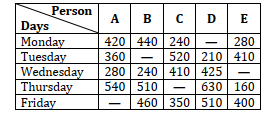Note: Some data are missing, calculate the missing data if required.

If average of items sold by D on Monday and Tuesday is 245. Then items sold by D on Monday is what percent of item sold by E on Friday ?

a
50%
b
None of these
c
60%
d
70%
e
75%View Answer
Explanation :

Items sold by D on Monday = 245 × 2 – 210

= 280

Required $\%=\frac{280}{400} \times 100=70 \%$

12.

If items sold by C on Thursday is average of items sold by C on Wednesday and Friday then find total items sold by C ?

a
None of these
b
1900
c
1700
d
1750
e
1850View Answer
Explanation :

Required total $=\left(\frac{410+350}{2}\right)+250+520+$

$410+350=1900$

13.

If total item sold by A and B including all fivedays is 2000 and 2200 respectively. Then item sold by B on Tuesday is what percent more/less than item sold by A on Friday ?

a
42.5%
b
23.5%
c
37.5%
d
32.25%
e
47%View Answer
Explanation :

Items sold by A on Friday = 2000 – (420 +360 + 280 + 540)

= 2000 – 1600 = 400

Items sold by B on Tuesday

= 2200 – (440 + 240 + 510 +460) = 550

Required $\%=\frac{(550-400)}{400} \times 100=37.5 \%$

14.

If ratio of items sold by B and C together on Thursday to items sold by C and E together on same day is 2 : 1. Then find item sold by C on Thursday ?

a
150
b
190
c
210
d
None of these
e
170View Answer
Explanation :

Let items sold by C on Thursday be x.

ATQ,

$\frac{510+x}{x+160}=\frac{2}{1}$

510 + x = 2x + 320

x = 190

15.

What is difference of item sold by A on Monday and Friday. If total item sold by A is 1800 ?

a
225
b
210
c
None of these
d
320
e
220View Answer
Explanation :

Item sold by A on Friday = 1800 – 1600= 200

Required difference = 420 – 200 = 220

16.  Directions(Q.16 to Q.25 ):

What will come in place of ’x’ in the following questions. Find the exact value.

$\frac{242}{6} \div \frac{3}{4}+x=31$

a
$\frac{-215}{9}$
b
$\frac{-225}{9}$
c
$\frac{-235}{9}$
d
$\frac{-205}{9}$
e
None of theseView Answer
Explanation :

$\frac{242}{6} \times \frac{4}{3}+\mathrm{x}=31$

or, $\frac{242 \times 2}{9}+\mathrm{x}=31$

or, $x=31-\frac{484}{9}$

or, $x=\frac{279-484}{9}=\frac{-205}{9}$

17.

4349.1 + 2256.4 – 1151.2 – 1244.3 = x

a
4321
b
4230
c
4210
d
4530
e
None of theseView Answer
Explanation :

6605.5 – 2395.5 = x

or, x = 4210

18.

$729 \times \frac{1}{9^{2}}-24 \div 3 \%$ of $10=\mathrm{x}$

a
-71
b
-70
c
-72
d
-76
e
None of theseView Answer
Explanation :

$729 \times \frac{1}{81}-24 \times \frac{100}{3 \times 10}=\mathrm{x}$

or, 9 – 80 = x

or, x = –71

19.

1728 ÷ 12³ – 11³ = x

a
-1330
b
-1142
c
-1342
d
-1332
e
None of theseView Answer
Explanation :

$1728 \times \frac{1}{12^{3}}-11^{3}=x$

or, $1728 \times \frac{1}{1728}-1331=\mathrm{x}$

or, x = –1330

20.

1089 × 101 + 36 × 44 = x

a
111573
b
111576
c
111546
d
111453
e
None of theseView Answer
Explanation :

1089 × (100 + 1) + (40 – 4) (40 + 4) = x

or, 1089 + 108900 + 40² – 4² = x

or, 109989 + 1600 – 16 = x

or, 111573 = x

21.

1001 × 1120 – 1120 × 999 + 55 = x

a
2295
b
2234
c
2245
d
2278
e
None of theseView Answer
Explanation :

1120 (1001 – 999) + 55 = x

or, 1120 × 2 + 55 = x

or, 2240 + 55 = x

or, x = 229

22.

$\frac{2}{7}$ of $343+\frac{512}{256}=\mathrm{x}$

a
100
b
90
c
80
d
110
e
None of theseView Answer
Explanation :

$\frac{2}{7} \times 343+2=x$

or, 98 + 2 = x

or, x = 100

23.

62 ÷ 31% of 200 + x = 4

a
2
b
4
c
3
d
5
e
None of theseView Answer
Explanation :

$62 \times \frac{100}{31 \times 200}+x=4$

or, 1 + x = 4 or, x = 3

24.

$\frac{3432}{4} \times \frac{5}{3}+168=\mathrm{x}$

a
1546
b
1432
c
1598
d
1234
e
None of theseView Answer
Explanation :

$\frac{3432}{4} \times \frac{5}{3}+168=\mathrm{x}$

or, $858 \times \frac{5}{3}+168=x$

or, 286 × 5 + 168 = x

or, 1430 + 168 = x

or, x = 1598

25.

$64 \times 198+\frac{340}{8} \times \frac{4}{17}=?$

a
12345
b
12435
c
12682
d
14567
e
None of theseView Answer
Explanation :

$64 \times(200-2)+\frac{20}{2}=x$

or, 12800 – 128 + 10 = x

or, x = 12682

26.  Directions(Q.26 to Q.30 ):

Find the value of question mark (?) in following number series:

10, 13, 18, 26, 38, ?

a
43
b
55
c
45
d
65
e
None of theseView Answer
Explanation :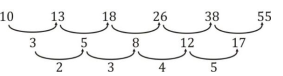27.

2, 7, 25, 105, 531, ?

a
3567
b
3241
c
2341
d
3193
e
None of theseView Answer
Explanation :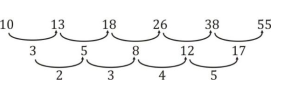28.

3, 4, 8, 17, 33, ?

a
58
b
45
c
54
d
47
e
None of theseView Answer
Explanation :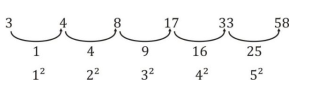29.

7, 7, 10, 18, 33, ?

a
57
b
54
c
65
d
34
e
None of theseView Answer
Explanation :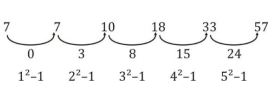30.

5, 7, 13, 25, 45, ?

a
45
b
75
c
65
d
86
e
None of theseView Answer
Explanation :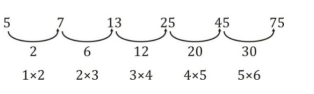31.  Directions(Q.31 to Q.35 ):

Study the passage carefully and answer the questions based on it.

Marks obtained by X in Physics is 160 which is 40% of total marks obtained by him. And marks obtained by Z in chemistry is ¾th of marks obtained by X in Physics. Ratio of total marks obtained by X to Z is 4 : 3. Marks obtained by Y in Maths is equal to marks obtained by Z in Chemistry. And total marks obtained by Y in Physics and Chemistry together is half of total marks obtained by X.

If ratio of marks obtained by Z in Physics and Maths is 2 : 1, then find average marks obtained by X and Z in Physics ?

a
None of these
b
150
c
160
d
120
e
140View Answer
Explanation :
Marks obtained X in Physics = 160 Total marks obtained by $\mathrm{X}=\frac{160}{40} \times 100=400$ Marks obtained by X in Chemistry & Maths together = 400 – 160 = 240 Total marks obtained by $\mathrm{Z}=\frac{400}{4} \times 3=300$ Marks obtained by $\mathrm{Z}$ in Chemistry $=\frac{3}{4} \times 160=120$ Marks obtained by Z in Physics and Maths = 300 – 120 = 180 Marks obtained by Y in Maths = 120 Marks obtained by Y in Physics and Chemistry Together $=\frac{400}{2}=200$ Total marks obtained by Y = 200 + 120 = 320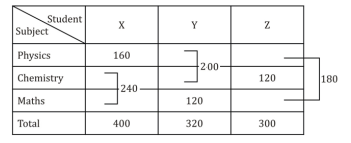Marks obtained by Z in Physics

= 180 × ⅔ = 120

Required average $=\frac{120+160}{2}=140$

32.

What is ratio of marks obtained by Z in Physics and Maths together to marks obtained by X in Chemistry and Physics together ?

a
2 : 5
b
4 : 3
c
cannot be determined
d
1 : 2
e
2 : 3View Answer
Explanation :
Marks obtained X in Physics = 160 Total marks obtained by $\mathrm{X}=\frac{160}{40} \times 100=400$ Marks obtained by X in Chemistry & Maths together = 400 – 160 = 240 Total marks obtained by $\mathrm{Z}=\frac{400}{4} \times 3=300$ Marks obtained by $\mathrm{Z}$ in Chemistry $=\frac{3}{4} \times 160=120$ Marks obtained by Z in Physics and Maths = 300 – 120 = 180 Marks obtained by Y in Maths = 120 Marks obtained by Y in Physics and Chemistry Together $=\frac{400}{2}=200$ Total marks obtained by Y = 200 + 120 = 320Cannot be determined

33.

If marks obtained by X in Maths is 50% more than marks obtained by him in Chemistry. Then find difference of marks obtained by X in Maths and marks obtained by Y in Maths ?

a
14
b
32
c
24
d
12
e
28View Answer
Explanation :
Marks obtained X in Physics = 160 Total marks obtained by $\mathrm{X}=\frac{160}{40} \times 100=400$ Marks obtained by X in Chemistry & Maths together = 400 – 160 = 240 Total marks obtained by $\mathrm{Z}=\frac{400}{4} \times 3=300$ Marks obtained by $\mathrm{Z}$ in Chemistry $=\frac{3}{4} \times 160=120$ Marks obtained by Z in Physics and Maths = 300 – 120 = 180 Marks obtained by Y in Maths = 120 Marks obtained by Y in Physics and Chemistry Together $=\frac{400}{2}=200$ Total marks obtained by Y = 200 + 120 = 320Let marks obtained by X in Chemistry be a.

ATQ,

$a+\frac{150}{100} a=240$

a = 96

Required difference = 96 × 1.5 – 120

= 144 – 120 = 24

34.

If Max. marks in each subject is 200. Then find percent of marks obtained by Y ?

a
42 ⅔%
b
61 ⅓%
c
None of these
d
53 ⅓%
e
47 ⅔%View Answer
Explanation :
Marks obtained X in Physics = 160 Total marks obtained by $\mathrm{X}=\frac{160}{40} \times 100=400$ Marks obtained by X in Chemistry & Maths together = 400 – 160 = 240 Total marks obtained by $\mathrm{Z}=\frac{400}{4} \times 3=300$ Marks obtained by $\mathrm{Z}$ in Chemistry $=\frac{3}{4} \times 160=120$ Marks obtained by Z in Physics and Maths = 300 – 120 = 180 Marks obtained by Y in Maths = 120 Marks obtained by Y in Physics and Chemistry Together $=\frac{400}{2}=200$ Total marks obtained by Y = 200 + 120 = 320Required $\%=\frac{320}{600} \times 100=531 / 3 \%$

35.

Marks obtained by Y in Physics and Chemistry together is what percent of total marks obtained by Z ?

a
None of these
b
50%
c
33 ⅓%
d
66 ⅔%
e
75%View Answer
Marks obtained X in Physics = 160 Total marks obtained by $\mathrm{X}=\frac{160}{40} \times 100=400$ Marks obtained by X in Chemistry & Maths together = 400 – 160 = 240 Total marks obtained by $\mathrm{Z}=\frac{400}{4} \times 3=300$ Marks obtained by $\mathrm{Z}$ in Chemistry $=\frac{3}{4} \times 160=120$ Marks obtained by Z in Physics and Maths = 300 – 120 = 180 Marks obtained by Y in Maths = 120 Marks obtained by Y in Physics and Chemistry Together $=\frac{400}{2}=200$ Total marks obtained by Y = 200 + 120 = 320Required $\%=\frac{200}{300} \times 100=662 / 3 \%$